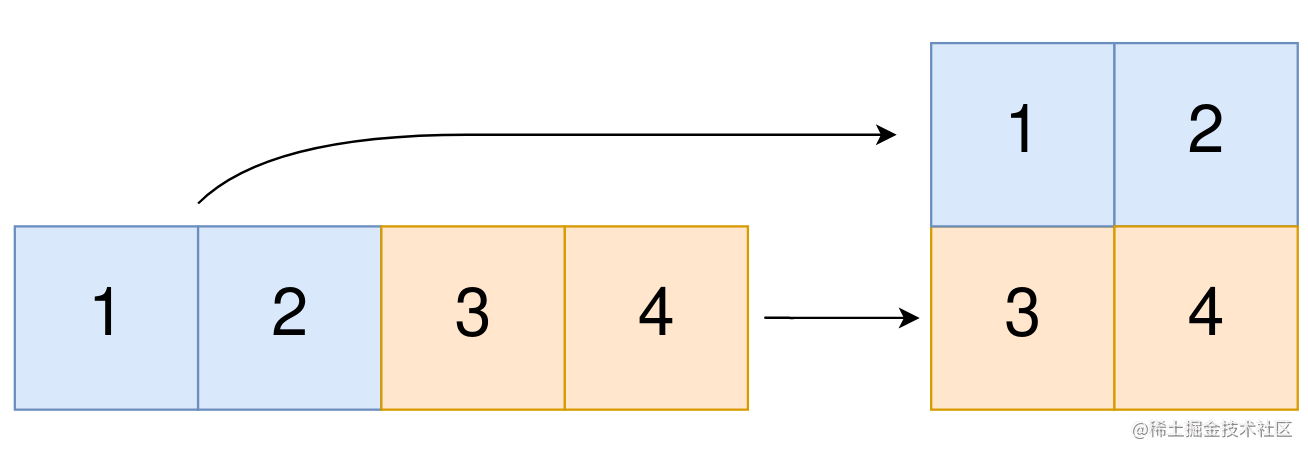leetcode 2022. Convert 1D Array Into 2D Array（python）

「这是我参与11月更文挑战的第7天，活动详情查看：2021最后一次更文挑战

描述

You are given a 0-indexed 1-dimensional (1D) integer array original, and two integers, m and n. You are tasked with creating a 2-dimensional (2D) array with m rows and n columns using all the elements from original.

The elements from indices 0 to n - 1 (inclusive) of original should form the first row of the constructed 2D array, the elements from indices n to 2 * n - 1 (inclusive) should form the second row of the constructed 2D array, and so on.

Return an m x n 2D array constructed according to the above procedure, or an empty 2D array if it is impossible.

Example 1:Input: original = [1,2,3,4], m = 2, n = 2
Output: [[1,2],[3,4]]
Explanation:
The constructed 2D array should contain 2 rows and 2 columns.
The first group of n=2 elements in original, [1,2], becomes the first row in the constructed 2D array.
The second group of n=2 elements in original, [3,4], becomes the second row in the constructed 2D array.

Example 2:

Input: original = [1,2,3], m = 1, n = 3
Output: [[1,2,3]]
Explanation:
The constructed 2D array should contain 1 row and 3 columns.
Put all three elements in original into the first row of the constructed 2D array.

Example 3:

Input: original = [1,2], m = 1, n = 1
Output: []
Explanation:
There are 2 elements in original.
It is impossible to fit 2 elements in a 1x1 2D array, so return an empty 2D array.

Example 4:

Input: original = , m = 1, n = 2
Output: []
Explanation:
There is 1 element in original.
It is impossible to make 1 element fill all the spots in a 1x2 2D array, so return an empty 2D array.

Note:

1 <= original.length <= 5 * 10^4
1 <= original[i] <= 10^5
1 <= m, n <= 4 * 104^

解析

• 先判断 m*n 如果和 original 长度不相等，直接返回空列表
• 否则初始化一个 result ，然后从左到右每次截取 n 个 original 中的元素加到 result 后面
• 遍历结束，返回 result

解答

class Solution(object):
def construct2DArray(self, original, m, n):
"""
:type original: List[int]
:type m: int
:type n: int
:rtype: List[List[int]]
"""
if m*n!=len(original) : return []
result = []
for i in range(m):
result.append(original[i*n:(i+1)*n])
return result

运行结果

Runtime: 884 ms, faster than 89.55% of Python online submissions for Convert 1D Array Into 2D Array.
Memory Usage: 22 MB, less than 50.87% of Python online submissions for Convert 1D Array Into 2D Array.